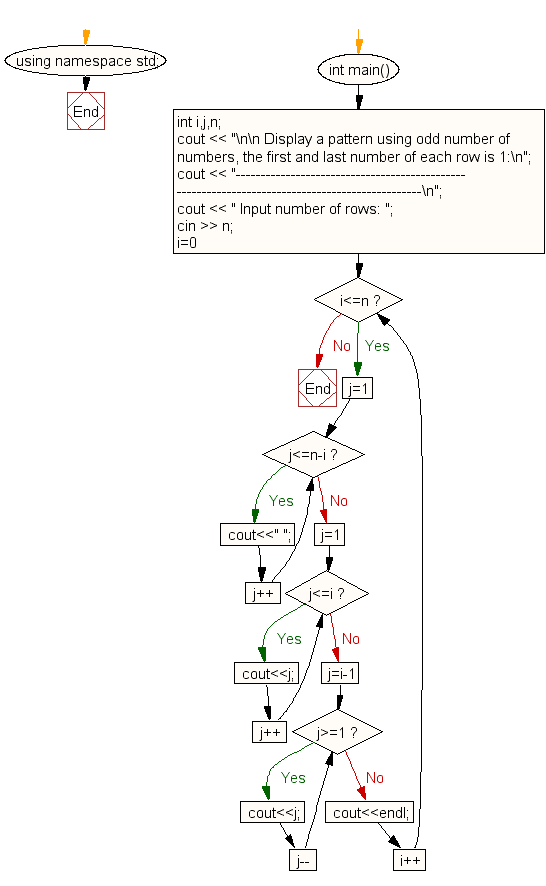﻿ C++ : Display a pattern for n number of rows using number

# C++ Exercises: Display such a pattern for n number of rows using number

## C++: For Loop Exercise-47 with Solution

Write a program in C++ to display such a pattern for n number of rows using numbers. Odd numbers will appear in each row. The first and last number of each row will be 1 and the middle column will be the row number.

Sample Solution:

C++ Code :

``````#include <iostream>
using namespace std;

int main()
{
int i,j,n;
cout << "\n\n Display a pattern using odd number of numbers, the first and last number of each row is 1:\n";
cout << "-----------------------------------------------------------------------------------------------\n";
cout << " Input number of rows: ";
cin >> n;
for(i=0;i<=n;i++)
{
/* print blank spaces */
for(j=1;j<=n-i;j++)
cout<<" ";
/* Display number in ascending order upto middle*/
for(j=1;j<=i;j++)
cout<<j;

/* Display  number in reverse order after middle */
for(j=i-1;j>=1;j--)
cout<<j;
cout<<endl;
}
}
``````

Sample Output:

``` Display a pattern using odd number of numbers, the first and last number of each row is 1:
-----------------------------------------------------------------------------------------------
Input number of rows: 5

1
121
12321
1234321
123454321
```

Flowchart:C++ Code Editor: